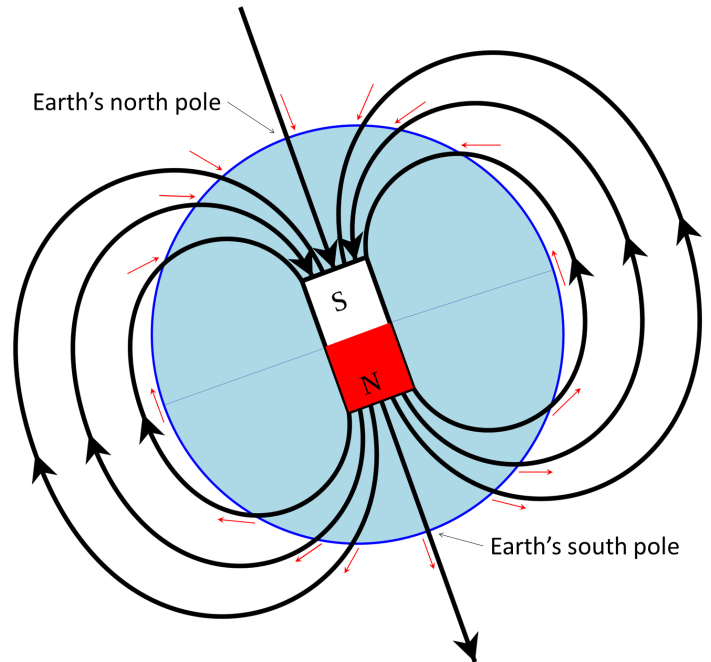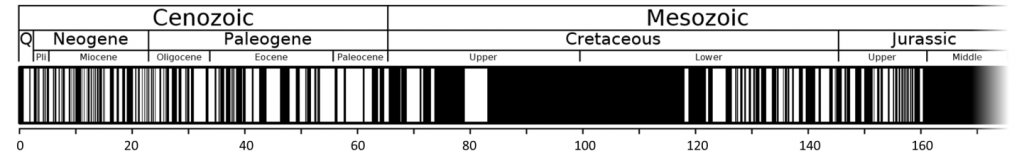# 9.3: Earth’s Magnetic Field

$$\newcommand{\vecs}{\overset { \rightharpoonup} {\mathbf{#1}} }$$ $$\newcommand{\vecd}{\overset{-\!-\!\rightharpoonup}{\vphantom{a}\smash {#1}}}$$$$\newcommand{\id}{\mathrm{id}}$$ $$\newcommand{\Span}{\mathrm{span}}$$ $$\newcommand{\kernel}{\mathrm{null}\,}$$ $$\newcommand{\range}{\mathrm{range}\,}$$ $$\newcommand{\RealPart}{\mathrm{Re}}$$ $$\newcommand{\ImaginaryPart}{\mathrm{Im}}$$ $$\newcommand{\Argument}{\mathrm{Arg}}$$ $$\newcommand{\norm}{\| #1 \|}$$ $$\newcommand{\inner}{\langle #1, #2 \rangle}$$ $$\newcommand{\Span}{\mathrm{span}}$$ $$\newcommand{\id}{\mathrm{id}}$$ $$\newcommand{\Span}{\mathrm{span}}$$ $$\newcommand{\kernel}{\mathrm{null}\,}$$ $$\newcommand{\range}{\mathrm{range}\,}$$ $$\newcommand{\RealPart}{\mathrm{Re}}$$ $$\newcommand{\ImaginaryPart}{\mathrm{Im}}$$ $$\newcommand{\Argument}{\mathrm{Arg}}$$ $$\newcommand{\norm}{\| #1 \|}$$ $$\newcommand{\inner}{\langle #1, #2 \rangle}$$ $$\newcommand{\Span}{\mathrm{span}}$$$$\newcommand{\AA}{\unicode[.8,0]{x212B}}$$

Heat is also being transferred from the solid inner core to the liquid outer core, and this leads to convection of the liquid iron of the outer core. Because iron is a metal and conducts electricity (even when molten), its motion generates a magnetic field.Figure $$\PageIndex{1}$$ Depiction of Earth’s magnetic field as a bar magnet coinciding with the core. The south pole of such a magnet points to Earth’s North Pole. The red arrows represent the orientation of the magnetic field at various locations on Earth’s surface.

Earth’s magnetic field is defined by the North and South Poles that align generally with the axis of rotation (Figure $$\PageIndex{1}$$). The lines of magnetic force flow into Earth in the northern hemisphere and out of Earth in the southern hemisphere. Because of the shape of the field lines, the magnetic force trends at different angles to the surface in different locations (red arrows of Figure $$\PageIndex{1}$$). At the North and South magnetic poles, the force is vertical. Anywhere near to the equator the force is horizontal, and everywhere in between, the magnetic force is at some intermediate angle to the surface. As we’ll see in Chapter 10, the variations in these orientations provide a critical piece of evidence to the understanding of continental drift as an aspect of plate tectonics.

Earth’s magnetic field is generated within the outer core by the convective movement of liquid iron, but as we discovered in Chapter 8, the magnetic field is not stable over geological time. For reasons that are not completely understood, the magnetic field decays periodically and then becomes re-established. When it does re-establish, it may be oriented the way it was before the decay, or it may be oriented with the reversed polarity. Over the past 250 Ma, there have been a few hundred magnetic field reversals, and their timing has been anything but regular. The shortest ones that geologists have been able to define lasted only a few thousand years, and the longest one was more than 30 million years, during the Cretaceous (Figure $$\PageIndex{2}$$).

##### Exercise 9.3 What would a magnetic dip meter tell you?

Regular compasses point only to the north magnetic pole, but if you have a magnetic dip meter you could also measure the angle of the magnetic field at your location in the up-and-down sense.

Using Figure $$\PageIndex{1}$$ as a guide, describe where you’d be on Earth if the vertical angles are as follows:

1. Up at a shallow angle
2. Parallel to the ground
3. Down at a steep angle
4. Straight down

See Appendix 3 for Exercise 9.3 answers.Figure $$\PageIndex{2}$$ Magnetic field reversal chronology for the past 170 Ma. The first 5 Ma of the magnetic chronology are shown in more detail in Figure $$\PageIndex{3}$$, although the time scale is in the opposite direction in that figure.

Changes in Earth’s magnetic field have been studied using a mathematical model, and reversals have been shown to take place when the model was run to simulate a period of several hundred thousand years. The fact that field reversals took place shows that the model is a reasonably accurate representation of the Earth. According to the lead author of the study, Gary Glatzmaier, of University of California at Santa Cruz: “Our solution shows how convection in the fluid outer core is continually trying to reverse the field but that the solid inner core inhibits magnetic reversals because the field in the inner core can only change on the much longer time scale of diffusion. Only once in many attempts is a reversal successful, which is probably the reason why the times between reversals of the Earth’s field are long and randomly distributed.” A depiction of Earth’s magnetic field lines during a stable period and during a reversal is shown in Figure $$\PageIndex{3}$$. To read more about these phenomena see Glatzmaier’s Geodynamo website.Figure $$\PageIndex{3}$$ Depiction of Earth’s magnetic field between reversals (left) and during a reversal (right). The lines represent magnetic field lines: blue where the field points toward Earth’s centre and yellow where it points away. The rotation axis of Earth is vertical, and the outline of the core is shown as a dashed white circle. 9.03: Earths Magnetic Field#fig9.3.3

## Image Descriptions9.03: Earths Magnetic Field#attachment_9609.03: Earths Magnetic Field#attachment_4799.03: Earths Magnetic Field#attachment_965

Figure $$\PageIndex{3}$$ image description: The earth’s magnetic fields is normally very uniform with the magnetic field pointing towards the earth in the north and away from the earth in the south. During a reversal, the Earth’s magnetic field becomes very convoluted. [Return to Figure $$\PageIndex{3}$$]

• Figure $$\PageIndex{1}$$: “Earths Magnetic Field Confusion” © TStein. Adapted by Steven Earle. CC BY-SA.
• Figure $$\PageIndex{2}$$: “Geomagnetic polarity 0-169 Ma” by Anomie. Adapted by Steven Earle. Public domain.
• Figure $$\PageIndex{3}$$: “Supercomputer models of Earth’s magnetic field” by NASA. Public domain.